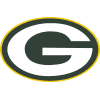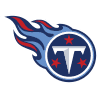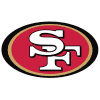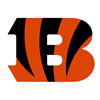DraftKings Super Bowl Odds
+350
3.5 to 1Packers
19.7% implied probability

+380
3.8 to 1Chiefs
18.5% implied probability

+500
5 to 1Bills
14.8% implied probability

+550
5.5 to 1Buccaneers
13.6% implied probability

+750
7.5 to 1Rams
10.4% implied probability

+850
8.5 to 1Titans
9.3% implied probability

+1000
10 to 149ers
8.1% implied probability

+1500
15 to 1Bengals
5.5% implied probability